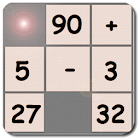Cronum Puzzle

All Android applications categories

All Android games categories# Cronum Puzzle

by: 75 8.4

8.4 Users
rating

## Screenshots

Description

Test your math skills! Cronum Puzzle is a logic game similar to the classic math puzzle. Consists of a square grid of eighty-cells (like Sudoku) filled with numbers and mathematical operators. The objective of the game is to find the number in the grid proposed in big on the right as a result of a mathematical operation like x + y, x - y, x * y or x / y. Identified the cells that give the result simply highlight them in the order of the operation by clicking each cell individually (number - operator - number). If the operation is correct it is proposed the next number to look for, otherwise the cells will go back to the original color. The gray cells are locked and can’t be selected. The operations can be vertical, horizontal and diagonal. The direction can be left / right, right / left, up / down or down / up! The operations are differentiated by level of difficulty. Easy level there are only operations of sum. A level Medium: addition and subtraction. A Hard level: addition, subtraction and multiplication. A Strong level: addition, subtraction, multiplication and division.

from 75 reviews

"Great"

8.4